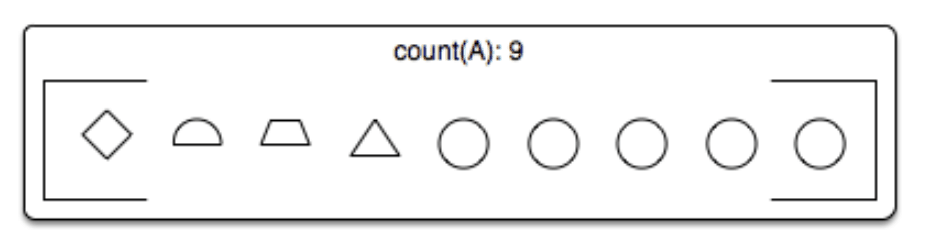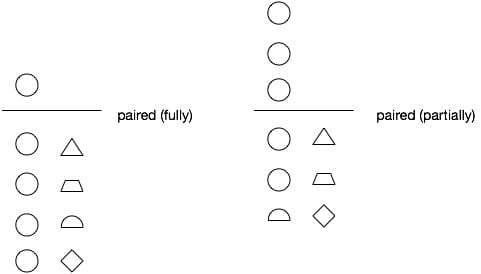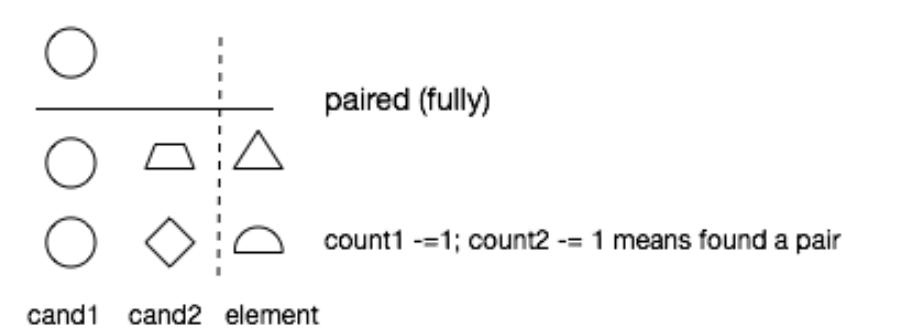# 229. Majority Element II

Reference: LeetCode
Difficulty: Medium

## Problem

Given an integer array of size n, find all elements that appear more than ⌊n/3⌋ times.

Note: The algorithm should run in linear time and in $O(1)$ space.

Example:

## Analysis

Methods:

1. HashMap

Time: $O(N)$
Space: $O(N)$

1. Boyer-Moore Majority Vote Algorithm

Let’s first go back to 169. Majority Element and try to think about the idea of pairing, which happens when count -= 1.

Suppose we have 9 items in an array:No matter in what order we select elements from the array, we can only get two results: Fully Paired and Partially Paired.So here comes the n/3 problem, we would only think about the fully pairing situation. If the over one third majority exists, it should be left after pairing.And why would we use three elements as a pair? Because it makes sure that in fully pairing the count of the majority element equals n/3.

More Examples:

Note:

• The ordering of if is very important. Though there are many branches, but each time only one could be executed.
• It is okay to initialize candidates with arbitrary numbers.

Time: $O(N)$
Space: $O(1)$

CommentJunhao Wang
a software engineering cat